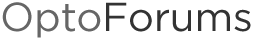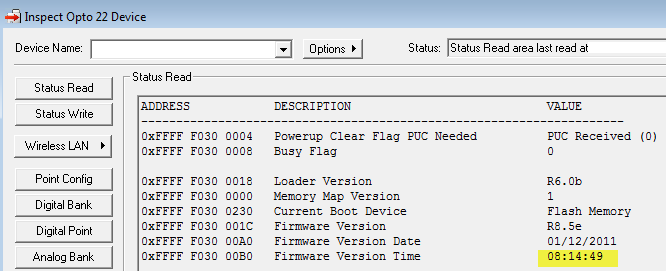Convert Integer 32 to Timestamp String

Fellow OptoFans,

I needed to convert a value in seconds into a nice HH:MM:SS string like you see below in PAC Manager. Alas, our PAC Control “String Commands” include many conversion options, like one called “Convert Integer 32 to IP Address String,” but none for a timestamp.

Of course there are many ways to solve this problem. Anyone else use something different? For example, I thought of filling the string with stars (like some of our string commands do) if you passed a string < 8 chars wide.

Have you solved this one, or something similar? Do share! I’ll attach a subroutine as well…

-OptoMary

// Get the hours:minutes:seconds from the total seconds

nTime_hours = nTotalSeconds/3600;
nTime_minutes = (nTotalSeconds - (nTime_hours * 3600))/60;
nTime_seconds = nTotalSeconds % 60;

NumberToStringField(nTime_hours, 2, sHH);
NumberToStringField(nTime_minutes, 2, sMM);
NumberToStringField(nTime_seconds, 2, sSS);

// Check for single digits and replace the leading space w/0
if (nTime_hours < 10) then // we have 1 digit
sHH = '0';
endif

if (nTime_minutes < 10) then // we have 1 digit
sMM = '0';
endif

if (nTime_seconds < 10) then // we have 1 digit
sSS = '0';
endif

sTimestamp = sHH + ":" + sMM + ":" + sSS;Hi All,

Here’s another one that comes up often enough AND, I thought I’d point out why you might consider upgrading to 9.1 (if you haven’t already). It includes the GetDateTime command, used in the example below to create a date/time stamp. Here’s an excerpt from the 9.1 PAC Control help:

Get Date & Time

Function: To read the date and time from a control engine’s real-time clock/calendar atomically (in a single transaction) and put each element an integer table.

Typical Use: This command assures that the date and time are retrieved on the same date.

Details: Reading the date and time separately could result in the following situation:
At 11:59:59 PM, a system operator reads the date—for example, April 1, 2011. Next, the operator reads the time., which now reads 00:00:02. The operator then stores the date and time, which reads as April 1, 2011 at 00:00:02. However, the actual date is now April 2, so the timestamp is a complete day behind. This error can also occur if you read the time first; reading atomically (in a single transaction) ensures you get a snapshot of the exact date and time you asked for.

Here’s some sample code to build a timestamp that looks something like this: “2012-01-24 16:45:22”

// Construct the timestamp, should look something like: "2012-01-24 16:45:22"
sTimestamp = "";

// Get the year/month/day in one transaction (so we avoid any problems w/values
// rolling over between individual reads)

// For the GetDateTime command: 0 = month, 1 = day of month, 2 = year,
// 3 = day of week, 4 = hours, 5 = minutes, 6 = seconds,
// 7 = milliseconds (unused)

// This command requires 9.1 or newer PAC Control and PAC firmware:
nStatus = GetDateTime(ntTimeTable);

// Year
NumberToString(ntTimeTable, sTemp);
while (GetStringLength(sTemp) < 4) sTemp = "0" + sTemp; wend // Pad with 0s
sTimestamp = sTemp;

// Month
NumberToString(ntTimeTable, sTemp);
while (GetStringLength(sTemp) < 2) sTemp = "0" + sTemp; wend // Pad with 0s
sTimestamp += "-";
sTimestamp += sTemp;

// Date
NumberToString(ntTimeTable, sTemp);
while (GetStringLength(sTemp) < 2) sTemp = "0" + sTemp; wend // Pad with 0s
sTimestamp += "-";
sTimestamp += sTemp;

sTimestamp += " "; // put a space after the date, before the time

// Hour
NumberToString(ntTimeTable, sTemp);
while (GetStringLength(sTemp) < 2) sTemp = "0" + sTemp; wend // Pad with 0s
sTimestamp += sTemp;

// Minute
NumberToString(ntTimeTable, sTemp);
while (GetStringLength(sTemp) < 2) sTemp = "0" + sTemp; wend // Pad with 0s
sTimestamp += ":";
sTimestamp += sTemp;

// Second
NumberToString(ntTimeTable, sTemp);
while (GetStringLength(sTemp) < 2) sTemp = "0" + sTemp; wend // Pad with 0s
sTimestamp += ":";
sTimestamp += sTemp;

I’ve attached a PAC Control 9.1 Basic subroutine too, in case anyone would like to add the “DateTimeToString” command into their 9.1-or-newer strategy.

-OptoMary

DateTimeToString.zip (1.56 KB)